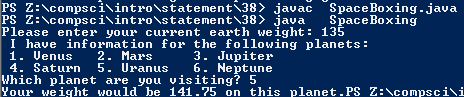# Assignemnt #38

## Code

```///Colin Hinton
///5th
///Space Boxing
///SpaceBoxing.java
///10/5/2015

import java.util.Scanner;

class SpaceBoxing
{
public static void main (String [] args)
{
Scanner keyboard = new Scanner(System.in);
int weight, planet;
Double venus, mars, jupiter, saturn, uranus, neptune;

weight = keyboard.nextInt();

System.out.println(" I have information for the following planets: ");
System.out.println(" 1. Venus   2. Mars     3. Jupiter");
System.out.println(" 4. Saturn  5. Uranus   6. Neptune");

System.out.print("Which planet are you visiting? ");
planet = keyboard.nextInt();

venus = ( weight * .78);
mars = ( weight * .39);
jupiter = ( weight * 2.65);
saturn = ( weight * 1.17);
uranus = ( weight * 1.05);
neptune = ( weight * 1.23);

if (planet == 1)
{
System.out.print("Your weight would be " + venus + " on this planet.");
}
else if (planet == 2)
{
System.out.print("Your weight would be " + mars + " on this planet.");
}
else if (planet == 3)
{
System.out.print("Your weight would be " + jupiter + " on this planet.");
}
else if (planet == 4)
{
System.out.print("Your weight would be " + saturn + " on this planet.");
}
else if (planet == 5)
{
System.out.print("Your weight would be " + uranus + " on this planet.");
}
else if (planet == 6)
{
System.out.print("Your weight would be " + neptune + " on this planet.");
}
}
}

```

### Picture of the output